# Help Replicating Excel FormulaHi,

We are trying to replicate the following set up and formulas from the below Excel snap shot into a Module. Currently the Module is set up where there are two list in the rows. In the Excel the formula for the Rollup is based on a calculation and we want to replicate that in Anaplan, but in Anaplan the Parent item automatically sums the children in the List. (attached is the Excel with the formulas in question highlighted in yellow).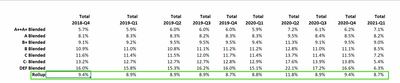Below is a screen shot of the Module we currently are using to try and create the Excel version.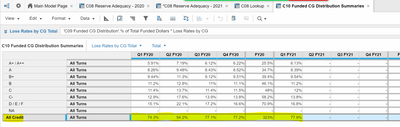Tagged:

•@neg177check out Ratio summary method:https://help.anaplan.com/9ab4e05a-1748-4027-****-525d5bdd5b22-Summary-Method#Ratio

To achive this in your case it can be used the following way: You have to create (in the same module) a separate line item with your calculation for Rollup and one more line item (number formatted) "1 as denominator"  with no dimensions an a  formula =1.

In ratio settings you have to put the Rollup formula in numerator and "1 as denominator" in denominator.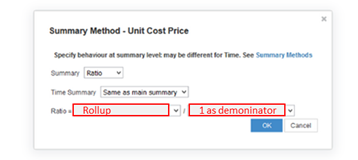This way your rollup calc will be devided by one. Which means - stays as is.

Cheers,

Kirill Kuznetsov

•Hi @neg177 ,

I would understand that you want to show 'weighted average' on the summary item.

You already have had the weights, so you multiple the targets number by them.

And sum the results and divide it by the sum of the weights.
Let's use the ratio summary method as @KirillKuznetsov  wrote.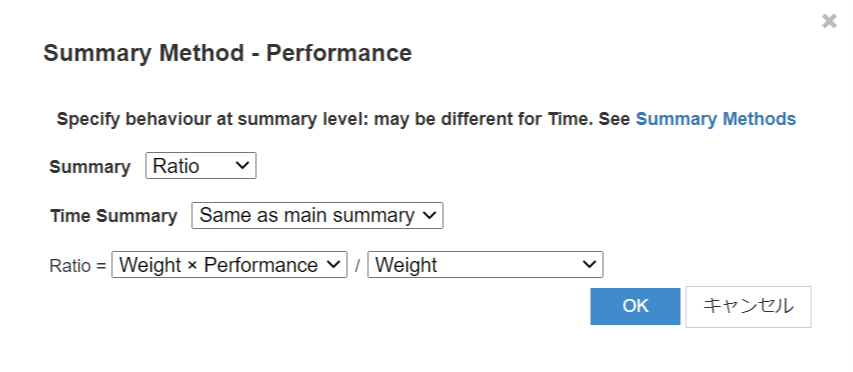In this situation, the sum of weight equals 1 (A below), but it is needless even to calculate weights, and you can use the amounts directly instead of the weights (B).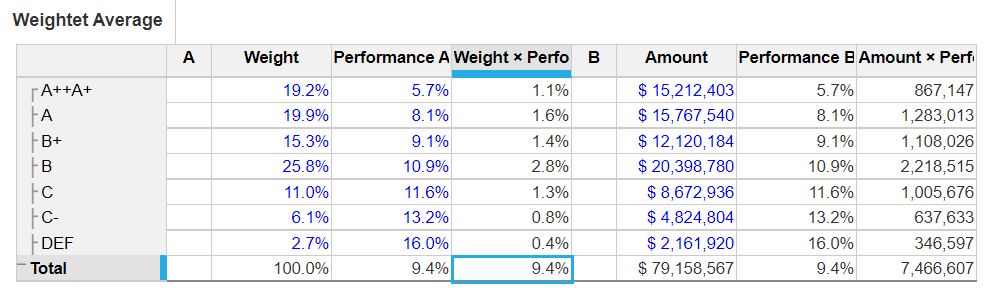I hope this helps.

Taichi